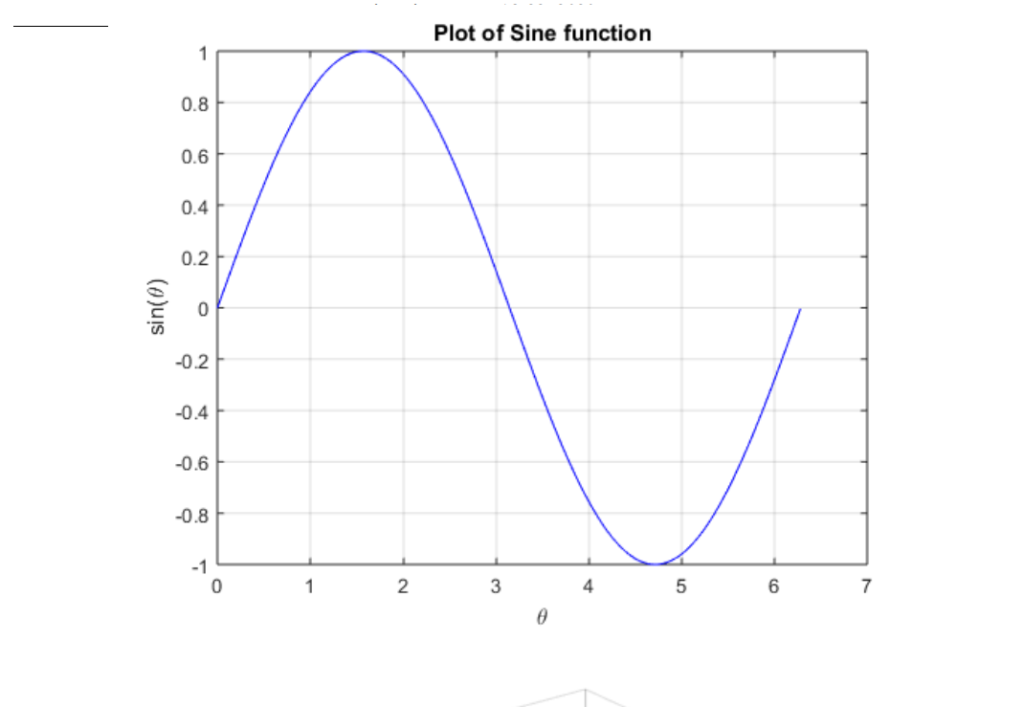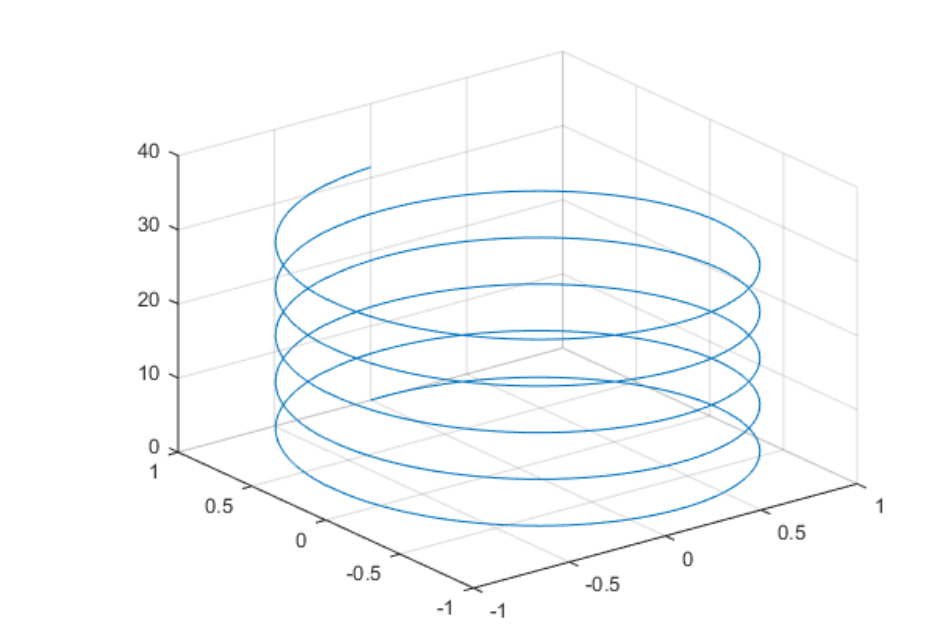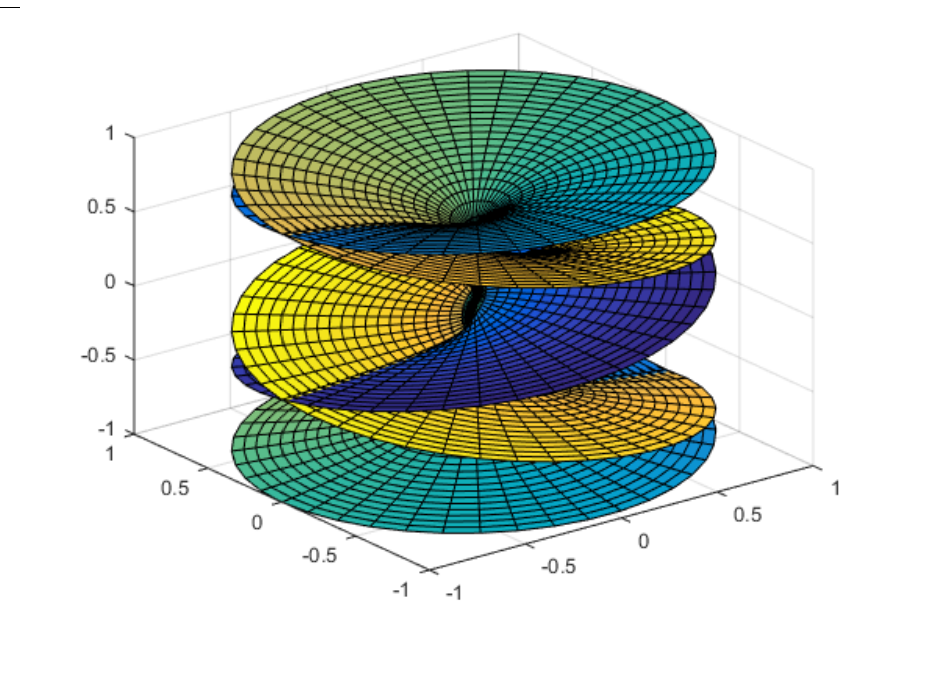# MATLAB – Plots in Detail

Prerequisite: Introduction to MATLAB
MATLAB is a powerful programming language, that can be used to draw various plots used in machine learning, deep learning, computer vision, and big data programming. Let us start with coding for plots in MATLAB.

Example 1: Let us first understand the simple plot :

 % angle from 0 to 2pi  theta = 0 : 0.01 : 2 * pi;      % sin function works on an array  f = sin(theta);  % 2D plot   plot(theta, f, 'b')     % label for X-axis   xlabel('\theta');     % label for Y-axis   ylabel('sin(\theta)');   title('Plot of Sine function')

Output :In the above code, the sine graph is plotted. Here as seen from the code we first define theta value of the sine plot, then build an array out of it and that function is finally plotted on the graph as shown in the output.

Example 2: Here we will plot the helix which is a 3-dimensional figure. As we all know from basic geometry that a helix is formed from the combination of the sine function and cosine function by declaring the value of theta and then iterating it over the same function. Try to visualize the output.

 % angle from 0 to 2pi  theta = 0:0.01:2*pi;      % sin function works on an array  f = sin(theta);      % 3D plot  t = 0 : pi / 50 : 10 * pi;     % open a new figure  figure      % 3D plot (helix)  plot3(sin(t), cos(t), t);

Output :Example 3: This is purely a complex plot, MATLAB being a highly visualization language for statics and plots. We have a scope to plot such types of graphs here. Here we have plotted the Riemann surface, in a 3D space. Try to visualize the output. Such types of complex functions can be plotted in MATLAB.

 % angle from 0 to 2pi  theta = 0 : 0.01 : 2 * pi;     % sin function works on an array  f = sin(theta);      t = 0 : pi / 50 : 10 * pi;     % open a new figure  figure      %  Riemann surfaces figure  cplxroot(5) % plots the Riemann Surface for w = z ^ (1 / 5)

Output :My Personal Notes arrow_drop_upEnthusiast Technical Content Writer

If you like GeeksforGeeks and would like to contribute, you can also write an article using contribute.geeksforgeeks.org or mail your article to contribute@geeksforgeeks.org. See your article appearing on the GeeksforGeeks main page and help other Geeks.

Please Improve this article if you find anything incorrect by clicking on the "Improve Article" button below.

Article Tags :

Be the First to upvote.

Please write to us at contribute@geeksforgeeks.org to report any issue with the above content.